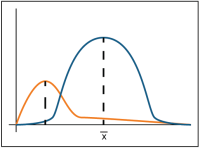## 5.6 Distributional TestsDistributional tests are commonly used to evaluate data distribution and to test data for normality. Many commonly applied statistical tests are parametricA statistical test that depends upon or assumes observations from a particular probability distribution or distributions (Unified Guidance). (i.e., they assume that the data follow a specific distribution, that they have a certain shape, and that the data can be described by a few parameters, such as the meanThe arithmetic average of a sample set that estimates the middle of a statistical distribution (Unified Guidance). (a measure of centrality) and standard deviation (a measure of spread).

Of the many different types of distributions used in statistics, the most commonly used are the normal distributionSymmetric distribution of data (bell-shaped curve), the most common distribution assumption in statistical analysis (Unified Guidance)., (also known as the bell curve) and distributions that can be transformed to a normal distribution (such as a lognormalA dataset that is not normally distributed (symmetric bell-shaped curve) but that can be transformed using a natural logarithm so that the data set can be evaluated using a normal-theory test (Unified Guidance). distribution). In addition, the gammaA gamma distribution or data set. A parametric unimodal distribution model commonly applied to groundwater data where the data set is left skewed and tied to zero. Very similar to Weibull and lognormal distributions; differences are in their tail behavior, and the gamma density has the second longest tail where its coefficient of variation is less than 1 (Unified Guidance; Gilbert 1987; Silva and Lisboa 2007). and Weibull distributions are used. The normal distribution (bell curve) is well known because of its common use in scholastic grading. This curve plots the frequency of occurrence on the vertical axis and the ordered values of interest, in our case, concentration, on the horizontal axis. If the data follow a normal distribution, most of the data concentrations are near the mean, or average, value and the likelihood of obtaining values away from the mean in either direction tapers off the further the concentration is from the mean.

Appendix A includes several case examples that provide examples of evaluating groundwater data with distributions.

### 5.6.1 Coefficients of Skewness and Variation

Because a normal, bell-shaped distribution is symmetric about the mean, normally distributed data will have zero skewnessA measure of asymmetry of a dataset (Unified Guidance).. Therefore, measuring the degree of skewness aids in evaluating data for normality and in evaluating the degree of non-normality. A coefficient of skewness greater than one indicates that the data are not normally distributed. Also, because of the symmetry of the normal curve, the medianThe 50th percentile of an ordered set of samples (Unified Guidance). value will be equal to the mean value. The coefficient of variation (the standard deviation divided by the mean) will also provide some measure of departure from normality. A coefficient of variation greater than one similarly indicates that the data are not normally distributed.

### 5.6.2 Kolmogorov-Smirnov Test

The Kolmogorov-Smirnov test (K-S test) is a common nonparametric goodness-of-fit test that compares the measured data distribution function with the normal distribution function (the mathematical model that generates the normal distribution). Thus, the K-S test compares the graphical curve (in this case, a cumulative fraction plot) of the measured data with that of the normal cumulative fraction plot. The method then calculates maximum distance between the two curves and estimates the p-valueIn hypothesis testing, the p-value gives an indication of the strength of the evidence against the null hypothesis, with smaller p-values indicating stronger evidence. If the p-value falls below the significance level of the test, the null hypothesis is rejected.. A p-value greater than the selected confidence levelDegree of confidence associated with a statistical estimate or test, denoted as (1 – alpha) (Unified Guidance). indicates that the data likely fit a normal distribution. A p-value below the selected confidence level indicates that the data do not fit a normal distribution.

### 5.6.3 Shapiro-Wilk Test

The Shapiro-Wilk test calculates an SW value. The SW value indicates whether a random sample comes from a normal distribution. If a data set is normally distributed, then a correlationAn estimate of the degree to which two sets of variables vary together, with no distinction between dependent and independent variables (USEPA 2013b). should exist between the ordered data and the normal distribution. Large values of SW indicate a strong correlation while small values of SW are evidence of departure from normally distributed data. This test has performed well in comparison studies with other goodness-of-fit tests.

### 5.6.4 Shapiro-Francia Normality Test

The Shapiro-Francia test is a simplified version of the Shapiro-Wilk test. The test is generally considered equivalent to Shapiro-Wilk test for large, independent samples. Like the Shapiro-Wilk test, the Shapiro-Francia test calculates an SF statistic to indicate whether a random sample comes from a normal distribution. If a data set is normally distributed, a correlation should exist between the ordered data and the z-scores taken from the normal distribution. Large values of SF indicate a strong correlation while small values of SF are evidence of departure from normally distributed data. The Shapiro-Francia test calculates an “SF” statistic. If the SF statistic exceeds the critical value, the test indicates that data likely fit a normal distribution. If the SF is less than the critical value, the test indicates that the data are not normally distributed. You may subsequently apply a data transformation, and retest for normality.

Publication Date: December 2013

Permission is granted to refer to or quote from this publication with the customary acknowledgment of the source (see suggested citation and disclaimer).

This web site is owned by ITRC.

1250 H Street, NW • Suite 850 • Washington, DC 20005

(202) 266-4933 • Email: [email protected]

ITRC is sponsored by the Environmental Council of the States.